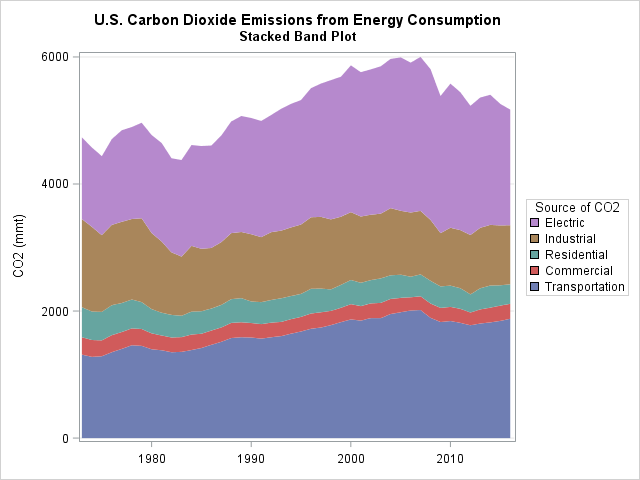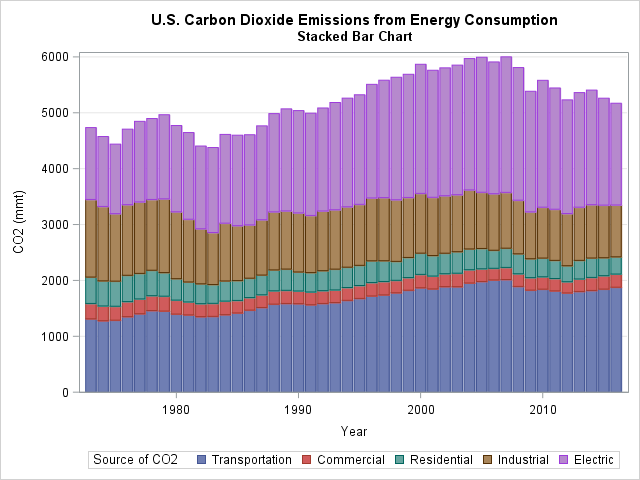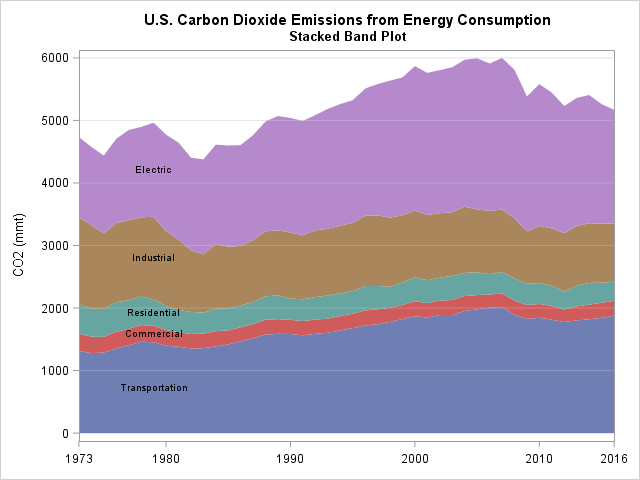This article shows how to construct a "stacked band plot" in SAS, as shown to the right. (Click to enlarge.) You are probably familiar with a stacked bar chart in which the cumulative amount of some quantity is displayed by stacking the contributions of several groups. A canonical example is a bar chart of revenue for a company in which the revenues for several regions (North America, Europe, Asia, and so forth) are stacked to show the total revenue. If you plot the revenue for several years, you get a stacked bar chart that shows how the total revenue changes over time, as well as the changes in the regional revenues. A stacked band plot is similar, but it is used when the horizontal variable is continuous rather than discrete.

### Create a stacked bar chart in SAS

A few years ago Sanjay and I each wrote about ways to create a stacked bar chart in SAS by using the SGPLOT procedure in SAS. Recently I created a stacked bar chart that spanned about 20 years of data. When I looked at it, I realized that as the number of categories (years) grows, the bars become a nuisance. The graph becomes cluttered with vertical bars that are not necessary to show the underlying trends in the data. At that point, it is better to switch to a continuous scale and use a stacked band plot.

This section shows how to create a stacked bar chart by using PROC SGPLOT. The next section shows how to create a stacked band chart.

For data, I decided to use the amount of carbon dioxide (CO2, in million metric tons) that is produced by several sources from 1973–2016. These data were plotted as a stacked bar chart by my friend Robert Allison. The data are originally in "wide form," with five variables that contain the data. For a stacked bar chart, you should convert the data to "long form," as follows. You can download the complete SAS program that creates the graphs in this article.

```/* 1. Original data is in "wide form": CO2 emission for five sources for each year. */ data CO2; informat Transportation Commercial Residential Industrial Electric COMMA5.; input Year Transportation Commercial Residential Industrial Electric; datalines; 2016 1,879 235 308 928 1,821 2015 1,845 240 321 942 1,913 2014 1,821 233 347 955 2,050 2013 1,803 223 333 952 2,050 ... more lines ... ;   /* 2. Convert from wide to long format */ proc sort data=CO2 out=Wide; by Year; /* sort X categories */ run;   proc transpose data=Wide out=Long(rename=(Col1=Value))/* name of new column that contains values */ name=Source; /* name of new column that contains categories */ by Year; /* original X var */ var Transportation Commercial Residential Industrial Electric; /* original Y vars */ run;```

For data in the long form, you can use the VBAR statement and the GROUPDISPLAY=STACK option in PROC SGPLOT to create a stacked bar chart, as follows:

```/* 3. create a stacked bar chart */ ods graphics / subpixel=on; title "U.S. Carbon Dioxide Emissions from Energy Consumption"; title2 "Stacked Bar Chart"; proc sgplot data=Long; vbar Year / response=Value group=Source groupdisplay=stack grouporder=data; xaxis type=linear thresholdmin=0; /* use TYPE=LINEAR so not every bar is labeled */ yaxis grid values=(0 to 6000 by 1000); label Source = "Source of CO2" Value = "CO2 (mmt)"; run;```The graph is not terrible, but it can be improved. The vertical lines are a distraction. With 43 years of data, the horizontal axis begins to lose its discrete nature. All those bars and the gaps between bars are cluttering the display. The next section shows how to create two new variables and use those variables to create a stacked band plot.

### Create a stacked band plot

To create a stacked bar chart with PROC SGPLOT, you must create two new variables. The cumValue variable will contain the cumulative amount of CO2 per year as you accumulate the amount for each group (Transportation, Commercial, Residential, and so forth). This will serve as the upper boundary of each band. The Previous variable will contain the lower boundary of each band. You can use the LAG function in Base SAS to easily obtain the previous cumulative value: Previous = lag(cumValue). When you create these variables, you need to initialize the value for the first group (Transportation). Use the BY YEAR statement and the FIRST.YEAR indicator variable to detect the beginning of each new value of the Year variable, as follows:

```/* 4. Accumulate the contributions of each group in the 'cumValue' variable. Add the lag(cumValue) variable and call it 'Previous'. Create a band plot for each group with LOWER=Previous and UPPER=cumValue. */ data Energy; set Long; by Year; if first.Year then cumValue=0; /* for each year, initialize cumulative amount to 0 */ cumValue + Value; Previous = lag(cumValue); if first.Year then Previous=0; /* for each year, initialize baseline value */ label Source = "Source of CO2" cumValue = "CO2 (mmt)"; run;   title2 "Stacked Band Plot"; proc sgplot data=Energy; band x=Year lower=Previous upper=cumValue / group=Source; xaxis display=(nolabel) thresholdmin=0; yaxis grid; keylegend / position=right sortorder=reverseauto; /* SAS 9.4M5: reverse legend order */ run;```

The graph appears at the top of this article. The vertical lines are gone. The height of a band shows the amount of CO2 that is produced by the corresponding source. The top of the highest band (Electric) shows the cumulative amount of CO2 that is produced by all sources. This kind of display makes it easy to see the cumulative total. If you want to see the trends for each individual source, a time series plot would be a better choice.

The graph uses a new feature of SAS 9.4M5. The KEYLEGEND statement now supports the SORTORDER=REVERSEAUTO option, which reverses the order of the legend elements. This option makes the legend match the bottom-to-top progression of the stacked bar chart. I also used the POSTITION= option to move the legend to the right side so that the legend is next to the color bands.

### Label the stacked bands directly

If you have very thin bands, a legend is probably the best way to associate colors with groups. However, for these data the bands are wide enough that you might want to display the name of each group on the bands. I tried several label positions (left, center, and right) and decided to display the group name near the left side of the graph. Since many people scan a graph from left to right, this causes the reader to see the labels early in the scanning process.

The following DATA step computes the positions for the labels. I use 1979 as the horizontal position and use the midpoint of the band as the vertical position. After you compute the positions for the labels, you can concatenate the data and the labels and use the TEXT statement to overlay the labels on the band plot, as follows:

```data Labels; set Energy; where Year = 1979; /* position labels at 1979 */ Label = Source; XPos = Year; YPos = (cumValue + Previous) / 2; keep Label XPos YPos; run;   data EnergyLabels; set Energy Labels; run;   title2 "Stacked Band Plot"; proc sgplot data=EnergyLabels noautolegend; band x=Year lower=Previous upper=cumValue / group=Source; refline 1000 to 6000 by 1000 / axis=y lineattrs=GraphGridLines transparency=0.75; text x=XPos y=Ypos text=Label; xaxis display=(nolabel) values=(1973, 1980 to 2010 by 10, 2016) offsetmin=0 offsetmax=0; yaxis grid values=(0 to 6000 by 1000) label="CO2 (mmt)"; run;```### Summary

In summary, you can use PROC SGPLOT to create a stacked band plot in SAS. A stacked band plot is similar to a stacked bar chart but presumes that the positions of the bars represent a continuous variable on a linear scale. For this example, the bars represented years. You can let PROC SGPLOT automatically place the legend along the bottom, but if you have SAS 9.4M5 you might want to move the legend to the right and use the SORTORDER=REVERSEAUTO option to reverse the legend order. Alternatively, you can display labels on the bands directly if there is sufficient room.

The post Create a stacked band plot in SAS appeared first on The DO Loop.